# Understanding Elementary Shapes Class 6 Notes Chapter 5

Understanding elementary shapes class 6 notes is one of the handiest resources for the students studying in CBSE Class 6. These notes are given in a simple and concise way and are easily understandable. These chapter 5 maths notes will not only help Class 6 students to learn various important topics from this chapter easily but will also help them to revise efficiently.

## Understanding Elementary Shapes Introduction

This chapter mostly deals with the measuring of line segments, about angles and its types, about triangles and their classifications, polygons, quadrilaterals, and solid shapes. All these topics are explained in detail below.

### Measuring Line Segments

A ruler or a divider is generally used to find the lengths of line segments. The length of a line segment can be defined as the distance between its endpoints.

#### For more information on Geometric Tools for Measurement, watch the below videoTo know more about Line Segment, visit here.

### Angles and Its Types

An angle is made up of any two rays which have the same endpoint or starting point. It can be better understood by the movement of clock-hands. When a clock hand moves, it forms an angle. To measure the angle, a protractor is used. It should be noted that a straight angle is 180 degrees while a right angle is 90 degrees.

Based on the degree, an angle can be classified into 3 main types:

• Acute angle: When an angle measure is less than a right angle, it is called an acute angle.
• Obtuse angle: When an angle measure more than a right angle but less than a straight angle, it is called an obtuse angle.
• Reflex angle: When an angle measure more than a straight angle, it is called a reflex angle.

It should be noted that two intersecting lines are perpendicular if the angle formed between them is 90 degrees.

#### For more information on Angles and Its Types, watch the below video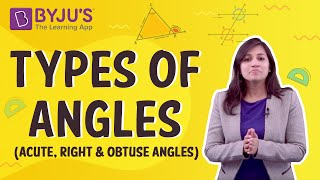To know more about Angles and Types of Angles, visit here.

### Triangle and its Types

A triangle is a closed figure having three sides and three interior angles. A triangle can be classified based on its length of sides and by its angles. The detailed classification of triangles is given below.

 Based on Triangle Name Description Based on its sides Scalene triangle All three sides are unequal. Isosceles triangle Any two sides are equal. Equilateral triangle All three sides are equal. Based on its angles Acute angled triangle All the angles are acute. Right-angled triangle Anyone angle is the right angle. Obtuse angled triangle Anyone angle is obtuse.

#### For more information on Triangles and Its Types, watch the below videoTo know more about Triangles, visit here.

### Polygons

Polygons are closed geometric shapes having at least 3 sides and 3 angles. Based on the number of sides, a polygon can be categorised into multiple types. Some of the most common polygons are-

 Polygon Name No. of Sides Triangle 3 Quadrilateral 4 Pentagon 5 Hexagon 6 Heptagon 7 Octagon 8 Nonagon 9 Decagon 10

To know more about Polygon, visit here.

As mentioned in the table above, a quadrilateral is one of the types of polygons having 4 sides and 4 angles. A quadrilateral can be categorised into 5 main types which are explained below.

 Quadrilateral Type Property Rhombus It has 4 sides of equal length. Square It is a rhombus with 4 right angles. Parallelogram It has two pairs of parallel sides. Rectangle It is a parallelogram of 4 right angles. Trapezium It has one pair of parallel sides.### Solid Shapes or 3D Shapes

A solid shape or three-dimensional shape (3D shape) can be defined as the objects which can be measured in three directions i.e. length, breadth, and height. Examples of 3d shapes are cylinder, cube, cuboid, sphere, etc. Check out three-dimensional shapes to learn more about them and to get acquainted with the terms related to them.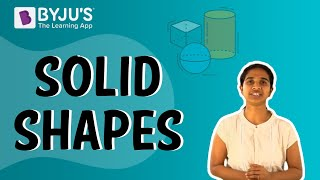To know more about 3D Shapes, visit here.

### Measuring Line Segments

The distance between the endpoints of a line segment is called its length.

⇒ Line segments can be measured by

• Comparison by observation
• Comparison by tracing
• Comparison using ruler and divider

### Positioning Error

To get the correct measure, the eye should be correctly positioned, just vertically above the mark. Errors can happen due to angular viewing.

## Perpendicular Lines

### Perpendicular Lines and Perpendicular Bisector

• When two lines intersect and the angle between them is a right angle, then the lines are said to be perpendicular.
• A perpendicular to a line segment that divides it exactly at the midpoint is called the perpendicular bisector.

## Classification of Triangles

• Triangles are those closed figures which have exactly three sides.
• Based on their sides and angles, they can be classified into different triangles.

### Types of Triangles Based on Lengths of Sides

Based upon the length of the sides, triangles are classified as:

• Scalene
• Isosceles
• Equilateral

#### For more information on Types Of Triangles Based on the Lengths of the Sides, watch the below video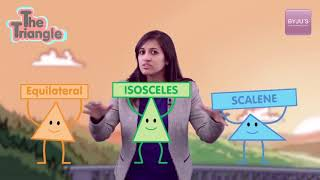### Types of Triangles Based on Angles

Based upon the measure of the angles, triangles are classified as:

• Acute-angled
• Obtuse-angled
• Right-angled

#### For more information on Types of Triangles Based on Angles, watch the below videoTo know more about Types of Triangles, visit here.

A quadrilateral is a polygon which has four sides.

Different quadrilaterals can be classified based on the lengths of the sides and angles.

### Rhombus

• A rhombus is a special type of parallelogram where all its sides are equal.
• The diagonals are perpendicular to each other. They also bisect the angles.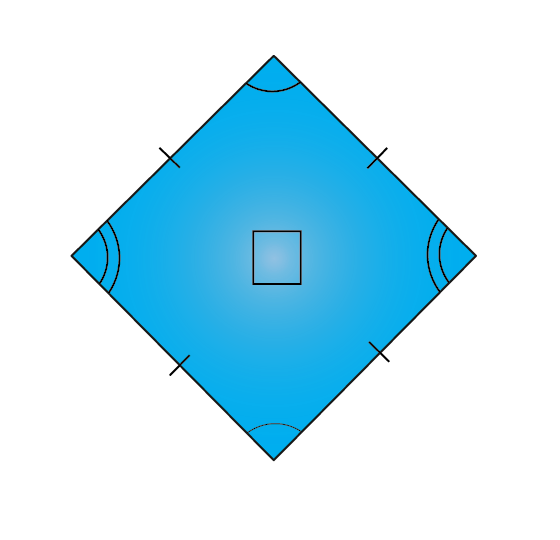To know more about Rhombus, visit here.

### Trapezium

• A trapezium is a quadrilateral where only two sides are parallel to each other.
• No sides, angles and diagonals are congruent.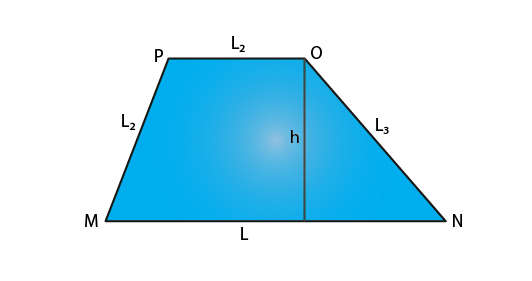To know more about Trapezium, visit here.

### Polygons

• A polygon is a closed figure made up of line segments in two-dimension.
• Polygons are classified based on the number of sides.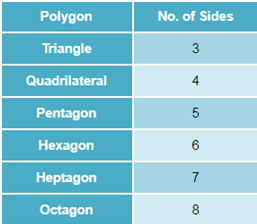To know more about Polygon, visit here.

### Rectangle

• A rectangle is a quadrilateral which has opposite sides equal and all angles are right angles.
• The diagonals are equal.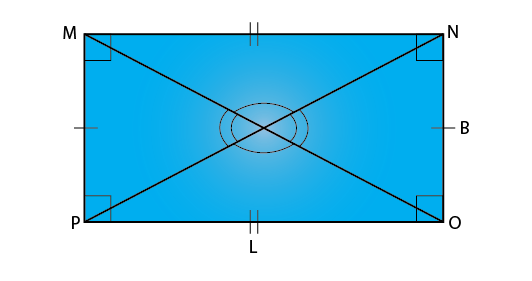To know more about Rectangle, visit here.

### Square

• A square is a quadrilateral which has all sides equal and all angles are right angles.
• The diagonals are equal and perpendicular to each other.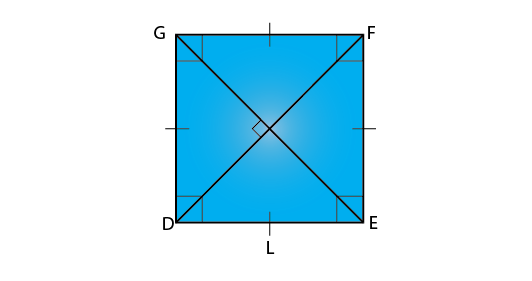To know more about Squares, visit here.

## Three-Dimensional Shapes

• Three-dimensional shapes are those shapes that can be projected on paper but not drawn on paper.
• These shapes are also called solids.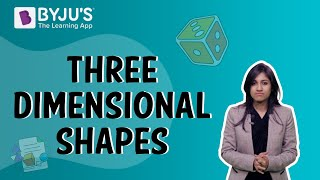### Faces, Edges and Vertices

• Each side of a three dimensional solid is called the face.
• Two faces meet at a line segment called an edge.
• Three edges meet at a point called a vertex.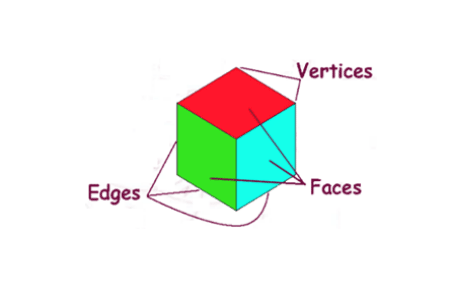### Special 3D Shapes

• Solids such as the prism and pyramid are considered as special 3D shapes since their structure is very unique.
• A prism with a triangular base is called a triangular prism.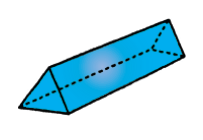A pyramid with a rectangular base is called a rectangular pyramid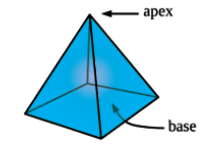## Angles

### Angles

The amount of rotation about the point of intersection of two planes (or lines) is called angle.

### Right, straight and complete angles

• Right angle is equal to 900.
• Straight angle is equal to 1800.
• Complete angle is one complete revolution or equal to 3600.

### Acute, Obtuse and Reflex Angles

• Acute angle is lesser than 900.
• Obtuse angle is greater than 900.
• Reflex angle is greater than 900.

### Tools of Construction

• Ruler and divider are used to measure lengths of line segments.
• A protractor is used to measure angles.

#### For more information on Tools of Construction, watch the below video### Measuring Angles

• Angles are measured in degrees.
• Angles are measured by using a protractor.

### Related Articles

 Types Of Triangles Quadrilateral Polygon Types Of Angles Line Segment NCERT Solutions Class 6 Maths Understanding Elementary Shapes

To get more such maths and science notes of Class 6, stay tuned with BYJU’S. Also, get several sample papers, questions papers and topic-wise study materials which can help to learn the class 6 topics more effectively.

## Frequently Asked Questions on CBSE Class 6 Maths Notes Chapter 5 Understanding Elementary Shapes

Q1

### What are the different types of triangles?

The different types of triangles are: equilateral, right isosceles, obtuse isosceles, acute isosceles, right scalene, obtuse scalene and acute scalene.

Q2

### What is a 3D shape?

3D shapes are shapes with three dimensions, such as width, height and depth. An example of a 3D shape is a prism or a sphere.

Q3

### What are geometric tools?

Geometric tools are the instruments used to draw different types of geometric shapes.